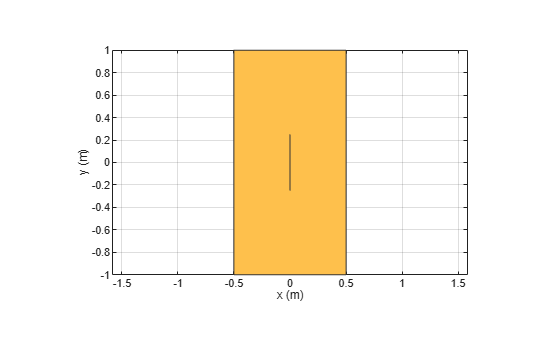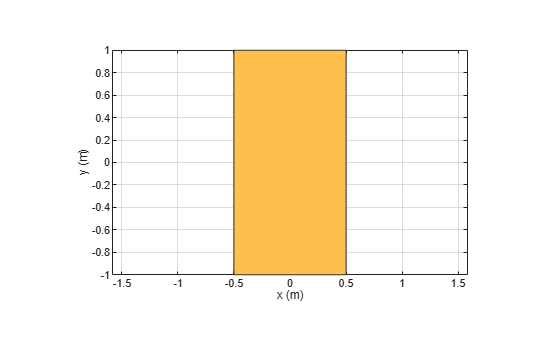# removeHoles

Remove holes from shape

## Syntax

``s = removeHoles(shapeobject,holetol)``

## Description

example

````s = removeHoles(shapeobject,holetol)` removes holes with area less than the provided tolerance from the shape.```

## Examples

collapse all

Create two rectangle shapes. Change the length and the center of orientation of the second rectangle to the values shown.

```rect1 = antenna.Rectangle; rect2 = antenna.Rectangle; rect2.Length = 1e-7; rect2.Width = 0.5```
```rect2 = Rectangle with properties: Name: 'myrectangle' Center: [0 0] Length: 1.0000e-07 Width: 0.5000 NumPoints: 2 ```

Subtract rectangle 1 from rectangle 2

`rect3 = rect1-rect2`
```rect3 = Polygon with properties: Name: 'mypolygon' Vertices: [9x3 double] ```
`show(rect3)`Remove holes from the new rectangle.

`rect4 = removeHoles(rect3,1e-6)`
```rect4 = Polygon with properties: Name: 'mypolygon' Vertices: [4x3 double] ```
`show(rect4)`## Input Arguments

collapse all

Antenna shape with sliver outlier, specified as `antenna.Circle`, `antenna.Rectangle`, `antenna.Polygon` objects, `antenna.Ellipse`, or the `shapes` function.

Data Types: `function`

Hole tolerance, specified as a nonnegative scalar.

Data Types: `double`

## Version History

Introduced in R2020b